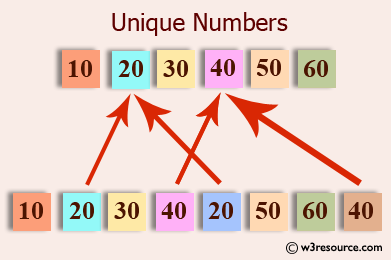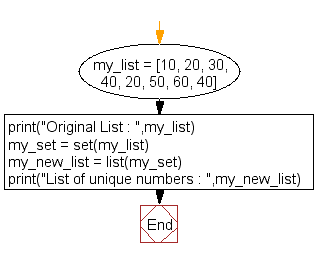﻿ Python: Get unique values from a list - w3resource# Python: Get unique values from a list

## Python List: Exercise - 29 with Solution

Write a Python program to get unique values from a list.Sample Solution:-

Python Code:

``````my_list = [10, 20, 30, 40, 20, 50, 60, 40]
print("Original List : ",my_list)
my_set = set(my_list)
my_new_list = list(my_set)
print("List of unique numbers : ",my_new_list)
```
```

Sample Output:

```Original List :  [10, 20, 30, 40, 20, 50, 60, 40]
List of unique numbers :  [40, 10, 50, 20, 60, 30]
```

Flowchart:## Visualize Python code execution:

The following tool visualize what the computer is doing step-by-step as it executes the said program:

Python Code Editor:

Have another way to solve this solution? Contribute your code (and comments) through Disqus.

What is the difficulty level of this exercise?

Test your Python skills with w3resource's quiz

﻿

## Python: Tips of the Day

Checks if the given number falls within the given range.

Example:

```def tips_range(n, start, end = 0):
return start <= n <= end if end >= start else end <= n <= start
print(tips_range(2, 4, 6))
print(tips_range(4, 8))
print(tips_range(1, 3, 5))
print(tips_range(1, 3))
```

Output:

```False
True
False
True
```